Next: The Probability Ranking Principle Up: Probabilistic information retrieval Previous: Probabilistic information retrieval   Contents   Index

# Review of basic probability theory

We hope that the reader has seen a little basic probability theory previously. We will give a very quick review; some references for further reading appear at the end of the chapter. A variable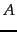represents an event (a subset of the space of possible outcomes). Equivalently, we can represent the subset via a random variable , which is a function from outcomes to real numbers; the subset is the domain over which the random variablehas a particular value. Often we will not know with certainty whether an event is true in the world. We can ask the probability of the event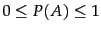. For two eventsand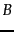, the joint event of both events occurring is described by the joint probability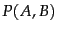. The conditional probability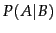expresses the probability of eventgiven that eventoccurred. The fundamental relationship between joint and conditional probabilities is given by the chain rule :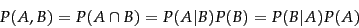(56)

Without making any assumptions, the probability of a joint event equals the probability of one of the events multiplied by the probability of the other event conditioned on knowing the first event happened.

Writing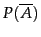for the complement of an event, we similarly have: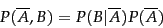(57)

Probability theory also has a partition rule , which says that if an eventcan be divided into an exhaustive set of disjoint subcases, then the probability ofis the sum of the probabilities of the subcases. A special case of this rule gives that: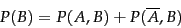(58)

From these we can derive Bayes' Rule for inverting conditional probabilities: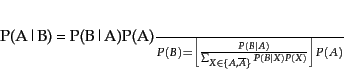(59)

This equation can also be thought of as a way of updating probabilities. We start off with an initial estimate of how likely the eventis when we do not have any other information; this is the prior probability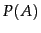. Bayes' rule lets us derive a posterior probabilityafter having seen the evidence, based on the likelihood ofoccurring in the two cases thatdoes or does not hold.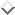Finally, it is often useful to talk about the odds of an event, which provide a kind of multiplier for how probabilities change: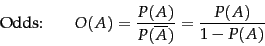(60)Next: The Probability Ranking Principle Up: Probabilistic information retrieval Previous: Probabilistic information retrieval   Contents   Index